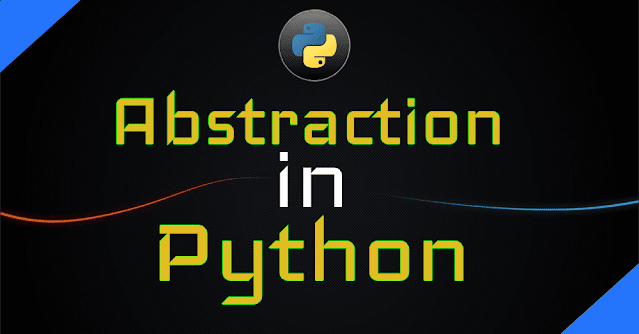# Data Abstraction and function overriding in PythonHello friends how are you, today in this blog i will teach you what is Data Abstraction, syntax of pointer , how Data Abstraction works and program using Data Abstraction in a very simple way.

Let's start

Data Abstraction

• Abstraction means Data hiding ,in other word we can say that in this type of programming essential data is shown to the user or outside class and unessential data is hidden.
• When we create an application then there are many elements are included but using Data Abstraction we can show that we want and we can hide that we don't want.
• In Python if we want to perform data hiding then it can be done by using double underscore(__) prefix with variables or functions then they can not be accessed outside that function.
``````
#defining base class
class Test1:
x=10
y=20
def myFun1(self):
print("This is function1")
class Test2(Test1):
def myFun2(self):
print("This is function2")
#Calling base class function and variable
print(self.x)
self.myFun1()
#creating object
obj=Test2()
obj.myFun2()
"""
***Output***
This is function2
10
This is function1
"""
``````
``````
#defining base class
class Test1:
__x=10
y=20
def myFun1(self):
print("This is function1")
class Test2(Test1):
def myFun2(self):
print("This is function2")
#Calling base class function and variable
print(self.x)
self.myFun1()
#creating object
obj=Test2()
obj.myFun2()
"""
***Output***
This is function2
Traceback (most recent call last):
AttributeError: 'Test2' object has no attribute 'x'
"""
``````

Function Overriding

• Function with same name and same parameters is called function overriding.
• It is not possible to make two function with same name and same parameters in a single class so we use two class.

``````
#defining base class
class Test1:
def myFun(self):
print("Base class Function")
#defining derived class
class Test2(Test1):
#override base class myFun
def myFun(self):
print("Derived class Function")
#creating object
obj=Test2()
obj.myFun()
"""
***Output***
Derived class Function
"""
``````
• Here Test 1 is Base class and Test2 is derived class,both classes contain a function myFun().
Request:-If you found this post helpful then let me know by your comment and share it with your friend.

If you want to ask a question or want to suggest then type your question or suggestion in comment box so that we could do something new for you all.

If you have not subscribed my website then please subscribe my website. Try to learn something new and teach something new to other.

Thanks.😊